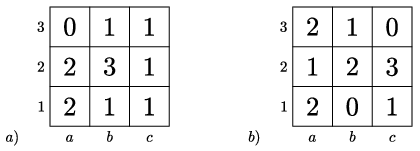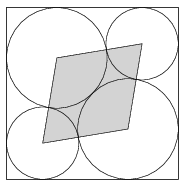Mathematical and Physical Journal
for High Schools
Issued by the MATFUND Foundation
 Already signed up? New to KöMaL?

# KöMaL Problems in Mathematics, March 2022

Show/hide problems of signs:## Problems with sign 'K'

Deadline expired on April 11, 2022.

K. 724. Julie cut a pizza into identical slices. Then she ate a few slices, but 3 slices remained. With a little calculation, she observed that she had eaten 3/4 of the whole pizza, plus 3/4 of a slice. How many slices were there?

(5 pont)

solution (in Hungarian), statistics

K. 725. We filled in the nine fields of a $\displaystyle 3\times3$ table one by one, according to the following rule: in each field, we entered the number of adjacent fields (i.e. fields sharing a common side with it) that had been filled in before. What was the order of the fields filled in? How many orders are possible? (Use the notation $\displaystyle a1, a2, \dots, c2, c3$ to refer to individual fields.)(5 pont)

solution (in Hungarian), statistics

K. 726. Arrange the numbers $\displaystyle 1, 2, 3, 4, \dots, 31, 32$ along the circumference of a circle such that the sum of any pair of adjacent numbers should be a perfect square. Explain your reasoning.

(5 pont)

solution (in Hungarian), statistics## Problems with sign 'K/C'

Deadline expired on April 11, 2022.

K/C. 727. On each field of an $\displaystyle n\times n$ table there is a coin, on all of them heads'' showing on top. In each move, we can turn over exactly three coins in any row or column, changing heads to tails and tails to heads. If $\displaystyle n>2$, is it possible to achieve with a sufficient number of moves that tails should show on top of each coin? Explain your answer.

(5 pont)

solution (in Hungarian), statistics

K/C. 728. We have 10 cards, with the numbers 1, 2, 3, 4, 5, 6, 7, 8, 9, 10 written on them. The cards are laid on the table in a random order in a row, and then we write the number of its position on each card (that is, the cards are numbered from 1 to 10). Thus there will be two numbers on each card. The two numbers on each card are multiplied together, and the products are all added up.

$\displaystyle a)$ What is the smallest possible value of the final sum?

$\displaystyle b)$ What is the largest possible value of the final sum?

(5 pont)

solution (in Hungarian), statistics## Problems with sign 'C'

Deadline expired on April 11, 2022.

C. 1709. The integers $\displaystyle a$ and $\displaystyle b$ are factors of 720, but $\displaystyle ab$ is not a factor of 720. How many such ordered pairs $\displaystyle (a;b)$ are there?

Proposed by S. Róka, Nyíregyháza

(5 pont)

solution (in Hungarian), statistics

C. 1710. Four circles are drawn in a unit square as shown in the figure. The two larger circles have the same size, and they are tangent to each other as well as to the sides of the square. The two smaller circles are also congruent, and they are also tangent to the sides of the square and to the larger circles. What is the area of the rhombus formed by the centres of the four circles?Proposed by M. E. Gáspár, Budapest

(5 pont)

solution (in Hungarian), statistics

C. 1711. Solve the equation

$\displaystyle \sqrt{x-1801}+\sqrt{y-1860}=2-\frac{1}{\sqrt{x-1801}},$

where $\displaystyle x$ and $\displaystyle y$ denote real numbers.

(5 pont)

solution (in Hungarian), statistics

C. 1712. The sides of a pentagon are all equal in length, and two of its angles are right angles. What may be the measures of the other three angles?

Proposed by G. Károlyi, Budajenő

(5 pont)

solution (in Hungarian), statistics

C. 1713. Let $\displaystyle x$ and $\displaystyle a$ denote real numbers such that $\displaystyle x+\frac{1}{x}=a$. Determine the value of $\displaystyle x^{13}+\frac{1}{x^{13}}$ as a function of $\displaystyle a$.

(5 pont)

solution (in Hungarian), statistics## Problems with sign 'B'

Deadline expired on April 11, 2022.

B. 5230. Points $\displaystyle C$ and $\displaystyle D$ lie on a semicircular arc of diameter $\displaystyle AB$. Let $\displaystyle A'$ and $\displaystyle B'$ denote the feet of the perpendiculars dropped to the line $\displaystyle CD$ from the points $\displaystyle A$ and $\displaystyle B$, respectively. Prove that the line segments $\displaystyle A'C$ and $\displaystyle B'D$ are equal in length.

Proposed by L. Surányi, Budapest

(3 pont)

solution (in Hungarian), statistics

B. 5231. Prove that

$\displaystyle \sum_{k=1}^n k \cdot 2^{k-1} = \sum_{k=1}^n 2^{n-k} \cdot (2^k-1).$

for all positive integers $\displaystyle n$.

(4 pont)

solution (in Hungarian), statistics

B. 5232. $\displaystyle P$ is an interior point lying on the median drawn from vertex $\displaystyle C$ of an acute-angled triangle $\displaystyle ABC$ such that $\displaystyle \angle APB =180^{\circ}-\angle ACB$. Show that the line $\displaystyle AB$ is tangent to the circle $\displaystyle APC$.

Proposed by Sz. Kocsis, Budapest

(4 pont)

solution (in Hungarian), statistics

B. 5233. The vertices of a regular hexagon are labelled $\displaystyle 1, 2, \ldots, 6$ in a random order. Then the absolute value of the difference of the labels of the adjacent endpoints is written on each side of the hexagon. Find the expected value of the sum of the six numbers written on the sides.

Proposed by J. Szoldatics, Budapest

(4 pont)

solution (in Hungarian), statistics

B. 5234. A positive integer $\displaystyle n$ is defined to be a mythical number if each of its divisors is 2 smaller than a prime number. For example, 15 is a mythical number. What is the largest possible number of divisors that a mythical number may have? Find all mythical numbers that have the maximum number of divisors.

Proposed by S. Róka, Nyíregyháza

(5 pont)

solution (in Hungarian), statistics

B. 5235. Show that all prime numbers greater than $\displaystyle 3$ that occur in the Fibonacci sequence are of the form $\displaystyle 4k+1$.

(5 pont)

solution (in Hungarian), statistics

B. 5236. Let $\displaystyle a$, $\displaystyle b$, $\displaystyle c$ denote positive real numbers such that $\displaystyle abc=1$. Show that

$\displaystyle a+a^2+a^3 + b + b^2 + b^3 + c+ c^2 + c^3 \le {(a^2+b^2+c^2)}^2.$

Proposed by M. Lovas, Budapest and M. Rozenberg, Israel

(6 pont)

solution (in Hungarian), statistics

B. 5237. In a triangle, $\displaystyle r$ denotes the inradius, $\displaystyle R$ is the circumradius, and $\displaystyle s$ denotes the semiperimeter. Prove that if $\displaystyle r+2R=s$ then the triangle is right angled.

Proposed by R. Fridrik, Szeged

(6 pont)

solution (in Hungarian), statistics## Problems with sign 'A'

Deadline expired on April 11, 2022.

A. 821. $\displaystyle a)$ Is it possible to find a function $\displaystyle f\colon \mathbb{N}^2\to \mathbb{N}$ such that for every function $\displaystyle g\colon \mathbb{N}\to \mathbb{N}$ and positive integer $\displaystyle m$ there exists $\displaystyle n \in \mathbb{N}$ such that set $\displaystyle \big\{k\in \mathbb{N} : f(n,k)=g(k)\big\}$ has at least $\displaystyle m$ elements?

$\displaystyle b)$ Is it possible to find a function $\displaystyle f\colon \mathbb{N}^2\to \mathbb{N}$ such that for every function $\displaystyle g\colon \mathbb{N}\to \mathbb{N}$ there exists $\displaystyle n \in \mathbb{N}$ such that set $\displaystyle \big\{k\in \mathbb{N} \colon f(n,k)=g(k)\big\}$ has an infinite number of elements?

(7 pont)

solution (in Hungarian), statistics

A. 822. Is it possible to find rational numbers $\displaystyle p$, $\displaystyle q$ and $\displaystyle r$ such that $\displaystyle p+q+r=0$ and $\displaystyle pqr=1$?

Proposed by Máté Weisz, Cambridge

(7 pont)

solution (in Hungarian), statistics

A. 823. For positive integers $\displaystyle n$ consider the lattice points $\displaystyle S_n=\big\{(x,y,z)\colon 1\le x\le n$, $\displaystyle 1\le y\le n$, $\displaystyle 1\le z\le n$, $\displaystyle x,y,z\in \mathbb{N}\big\}$. Is it possible to find a positive integer $\displaystyle n$ for which it is possible to choose more than $\displaystyle n\sqrt{n}$ lattice points from $\displaystyle S_n$ such that for any two chosen lattice points at least two of the coordinates of one is strictly greater than the corresponding coordinates of the other?

Proposed by Endre Csóka, Budapest

(7 pont)

solution (in Hungarian), statistics